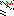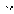# MINLPLib

### A Library of Mixed-Integer and Continuous Nonlinear Programming Instances

#### Instance st_e30

 Formatsⓘ ams gms lp mod nl osil pip py Primal Bounds (infeas ≤ 1e-08)ⓘ -1.58113883 p1 ( gdx sol ) (infeas: 1e-12) Other points (infeas > 1e-08)ⓘ Dual Boundsⓘ -1.58113890 (ANTIGONE)-1.58113884 (BARON)-1.58113883 (COUENNE)-1.58113965 (GUROBI)-1.58113883 (LINDO)-1.58113894 (SCIP) Referencesⓘ Tawarmalani, M and Sahinidis, N V, Convexification and Global Optimization in Continuous and Mixed-Integer Nonlinear Programming: Theory, Algorithms, Software, and Applications, Kluwer, 2002.Marin, R, Points of location on a fixture, 1998. Personal Communication. Sourceⓘ BARON book instance misc/e30 Added to libraryⓘ 03 Sep 2002 Problem typeⓘ QCP #Variablesⓘ 14 #Binary Variablesⓘ 0 #Integer Variablesⓘ 0 #Nonlinear Variablesⓘ 7 #Nonlinear Binary Variablesⓘ 0 #Nonlinear Integer Variablesⓘ 0 Objective Senseⓘ min Objective typeⓘ linear Objective curvatureⓘ linear #Nonzeros in Objectiveⓘ 1 #Nonlinear Nonzeros in Objectiveⓘ 0 #Constraintsⓘ 15 #Linear Constraintsⓘ 10 #Quadratic Constraintsⓘ 5 #Polynomial Constraintsⓘ 0 #Signomial Constraintsⓘ 0 #General Nonlinear Constraintsⓘ 0 Operands in Gen. Nonlin. Functionsⓘ Constraints curvatureⓘ indefinite #Nonzeros in Jacobianⓘ 42 #Nonlinear Nonzeros in Jacobianⓘ 14 #Nonzeros in (Upper-Left) Hessian of Lagrangianⓘ 14 #Nonzeros in Diagonal of Hessian of Lagrangianⓘ 2 #Blocks in Hessian of Lagrangianⓘ 2 Minimal blocksize in Hessian of Lagrangianⓘ 3 Maximal blocksize in Hessian of Lagrangianⓘ 4 Average blocksize in Hessian of Lagrangianⓘ 3.5 #Semicontinuitiesⓘ 0 #Nonlinear Semicontinuitiesⓘ 0 #SOS type 1ⓘ 0 #SOS type 2ⓘ 0 Minimal coefficientⓘ 1.0000e+00 Maximal coefficientⓘ 2.0000e+00 Infeasibility of initial pointⓘ 1 Sparsity JacobianⓘSparsity Hessian of Lagrangianⓘ```\$offlisting
*
*  Equation counts
*      Total        E        G        L        N        X        C        B
*         16        8        3        5        0        0        0        0
*
*  Variable counts
*                   x        b        i      s1s      s2s       sc       si
*      Total     cont   binary  integer     sos1     sos2    scont     sint
*         15       15        0        0        0        0        0        0
*  FX      0
*
*  Nonzero counts
*      Total    const       NL      DLL
*         44       30       14        0
*
*  Solve m using NLP minimizing objvar;

Variables  x1,x2,x3,x4,x5,x6,x7,x8,x9,x10,x11,x12,x13,x14,objvar;

Positive Variables  x11,x12,x13,x14;

Equations  e1,e2,e3,e4,e5,e6,e7,e8,e9,e10,e11,e12,e13,e14,e15,e16;

e1.. -x12*x7 - x1 + x3 =E= 0;

e2.. -x12*x8 - x2 + x4 =E= 0;

e3.. (-x13*x7) - x11*x9 - x1 + x5 =E= 0;

e4.. (-x13*x8) - x11*x10 - x2 + x6 =E= 0;

e5.. sqr(x7) + sqr(x8) =E= 1;

e6..    x8 + x9 =E= 0;

e7..  - x7 + x10 =E= 0;

e8..  - x12 + x14 =L= 0;

e9..  - x11 + x14 =L= 0;

e10..    2*x1 + x2 =G= -1;

e11..    2*x3 + x4 =G= -1;

e12..    2*x5 + x6 =G= -1;

e13..    x1 + x2 =L= 1;

e14..    x3 + x4 =L= 1;

e15..    x5 + x6 =L= 1;

e16..    x14 + objvar =E= 0;

* set non-default bounds
x1.lo = -1; x1.up = 1;
x2.lo = -1; x2.up = 1;
x3.lo = -1; x3.up = 1;
x4.lo = -1; x4.up = 1;
x5.lo = -1; x5.up = 1;
x6.lo = -1; x6.up = 1;
x7.lo = -1; x7.up = 1;
x8.lo = -1; x8.up = 1;
x9.lo = -1; x9.up = 1;
x10.lo = -1; x10.up = 1;
x11.up = 3;
x12.up = 3;
x13.up = 3;
x14.up = 3;

Model m / all /;

m.limrow=0; m.limcol=0;
m.tolproj=0.0;

\$if NOT '%gams.u1%' == '' \$include '%gams.u1%'

\$if not set NLP \$set NLP NLP
Solve m using %NLP% minimizing objvar;

```

Last updated: 2023-06-01 Git hash: fdbb0df7